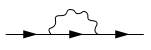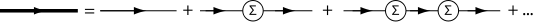# Self-energy

Self-energy

In theoretical physics and quantum field theory a particle's self-energy Σ represents the contribution to the particle's energy, or effective mass, due to interactions between the particle and the system it is part of. For example, in electrostatics the self-energy of a given charge distribution is the energy required to assemble the distribution by bringing in the constituent charges from infinity, where the electric force goes to zero. In a condensed matter context relevant to electrons moving in a material, the self-energy represents the potential felt by the electron due to the surrounding medium's interactions with it: for example, the fact that electrons repel each other means that a moving electron polarizes (causes to displace) the electrons in its vicinity and this in turn changes the potential the moving electron feels; these and other effects are included in the self-energy.

Mathematically, this energy is equal to the so-called on-the-mass-shell value of the proper self-energy operator (or proper mass operator) in the momentum-energy representation (more precisely, to$\hbar$ times this value). In this, or other representations (such as the space-time representation), the self-energy is pictorially (and economically) represented by means of Feynman diagrams, such as the one shown below. In this particular diagram, the three arrowed straight lines represent particles, or particle propagators, and the wavy line a particle-particle interaction; removing (or amputating) the left-most and the right-most straight lines in the diagram shown below (these so-called external lines correspond to prescribed values for, for instance, momentum and energy, or four-momentum), one retains a contribution to the self-energy operator (in, for instance, the momentum-energy representation). Using a small number of simple rules, each Feynman diagram can be readily expressed in its corresponding algebraic form.

In general, the on-the-mass-shell value of the self-energy operator in the momentum-energy representation is complex (see complex number). In such cases, it is the real part of this self-energy that is identified with the physical self-energy (referred to above as particle's self-energy); the inverse of the imaginary part is a measure for the lifetime of the particle under investigation. For clarity, elementary excitations, or dressed particles (see quasi-particle), in interacting systems are distinct from stable particles in vacuum; their state functions consist of complicated superpositions of the eigenstates of the underlying many-particle system, which only, if at all, momentarily behave like those specific to isolated particles; the above-mentioned lifetime is the time over which a dressed particle behaves as if it were a single particle with well-defined momentum and energy.

The self-energy operator (often denoted by$\Sigma_{}^{}$, and less frequently by$M_{}^{}$) is related to the bare and dressed propagators (often denoted by$G_0^{}$ and$G_{}^{}$ respectively) via the Dyson equation (named after Freeman John Dyson):$G = G_0^{} + G_0 \Sigma G$.

Multiplying on the left by the inverse$G_0^{-1}$ of the operator G0 and on the right by G − 1 yields$\Sigma = G_0^{-1} - G^{-1}$.The photon and gluon do not get a mass through renormalization because gauge symmetry protects them from getting a mass. This is a consequence of the Ward identity. The W-boson and the Z-boson get their masses through the Higgs mechanism; they do undergo mass renormalization through the renormalization of the electroweak theory.

Neutral particles with internal quantum numbers can mix with each other through virtual pair production. The primary example of this phenomenon is the mixing of neutral kaons. Under appropriate simplifying assumptions this can be described without quantum field theory.

In chemistry, the self-energy or Born energy of an ion is the energy associated with the field of the ion itself.

In solid state and condensed-matter physics self-energies and a myriad of related Quasiparticle properties are calculated by Green's function methods and Green's function (many-body theory) of interacting low-energy excitations on the basis of electronic band structure calculations.

Wikimedia Foundation. 2010.

### Look at other dictionaries:

• self energy — savoji energija statusas T sritis fizika atitikmenys: angl. intrinsic energy; self energy vok. Eigenenergie, f; Selbstenergie, f rus. собственная энергия, f pranc. auto énergie, f; énergie propre, f; self énergie, f …   Fizikos terminų žodynas

• self-energy — (ˈ) ̷ ̷| ̷ ̷ ̷ ̷ ̷ ̷ noun : energy that is generated in or by itself …   Useful english dictionary

• self-energy — n. * * * …   Universalium

• Self-Generator —   A plant whose primary product is not electric power, but does generate electricity for its own use or for sale on the grid; for example, industrial combined heat and power plants.   U.S. Dept. of Energy, Energy Information Administration s… …   Energy terms

• Self-generation —   A generation facility dedicated to serving a particular retail customer,usually located on the customer s premises. The facility may either be owned directly by the retail customer or owned by a third party with a contractual arrangement to… …   Energy terms

• Self-service wheeling —   Primarily an accounting policy comparable to net billing or running the meter backwards. An entity owns generation that produces excess electricity at one site, that is used at another site(s) owned by the same entity. It is given billing… …   Energy terms

• self-énergie — savoji energija statusas T sritis fizika atitikmenys: angl. intrinsic energy; self energy vok. Eigenenergie, f; Selbstenergie, f rus. собственная энергия, f pranc. auto énergie, f; énergie propre, f; self énergie, f …   Fizikos terminų žodynas

• Self-creation cosmology — (SCC) theories are gravitational theories in which the mass of the universe is created out of its self contained gravitational and scalar fields, as opposed to the theory of continuous creation cosmology or the steady state theory which depend on …   Wikipedia

• Energy policy — is the manner in which a given entity (often governmental) has decided to address issues of energy development including energy production, distribution and consumption. The attributes of energy policy may include legislation, international… …   Wikipedia

• Self-organization — is a process of attraction and repulsion in which the internal organization of a system, normally an open system, increases in complexity without being guided or managed by an outside source. Self organizing systems typically (though not always)… …   Wikipedia